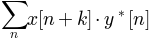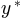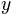You are reading an old version of the documentation (v3.0.0). For the latest version see https://matplotlib.org/stable/api/_as_gen/matplotlib.pyplot.xcorr.html

# matplotlib.pyplot.xcorr¶

`matplotlib.pyplot.``xcorr`(x, y, normed=True, detrend=<function detrend_none>, usevlines=True, maxlags=10, *, data=None, **kwargs)[source]

Plot the cross correlation between x and y.

The correlation with lag k is defined as, whereis the complex conjugate of.

Parameters: x : sequence of scalars of length n y : sequence of scalars of length n detrend : callable, optional, default: `mlab.detrend_none` x is detrended by the detrend callable. Default is no normalization. normed : bool, optional, default: True If `True`, input vectors are normalised to unit length. usevlines : bool, optional, default: True If `True`, `Axes.vlines` is used to plot the vertical lines from the origin to the acorr. Otherwise, `Axes.plot` is used. maxlags : int, optional Number of lags to show. If None, will return all `2 * len(x) - 1` lags. Default is 10. lags : array (length `2*maxlags+1`) lag vector. c : array (length `2*maxlags+1`) auto correlation vector. line : `Artist` added to the axes of the correlation `LineCollection` if usevlines is True `Line2D` if usevlines is False b : `Line2D` or None Horizontal line at 0 if usevlines is True None usevlines is False linestyle : `Line2D` property, optional Only used if usevlines is `False`. marker : string, optional Default is 'o'.

Notes

The cross correlation is performed with `numpy.correlate()` with `mode = 2`.

Note

In addition to the above described arguments, this function can take a data keyword argument. If such a data argument is given, the following arguments are replaced by data[<arg>]:

• All arguments with the following names: 'x', 'y'.

Objects passed as data must support item access (`data[<arg>]`) and membership test (`<arg> in data`).